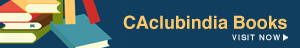# Understanding Foreign Exchange - Part 3

Aravind.. , 16 August 2011

-Series of Articles on Foreign Exchange-

When speaking about foreign currency market, we have come across two terms – spot rate and forward rate. Normally forward rates are for fixed periods – one month, three months and six months. Usually, forward rates give us a some idea about the expectations of the strength that a currency could have against another foreign currency. For instance lets take up this example,

Spot rate :                        INR/\$ = 48.75-49.25

One month fwd:              INR/\$ = 48.90-49.45

From the above example we can infer that an USD can be bought for INR 49.25 now and it can be bought at INR 49.45, a month later. From this we can understand that USD has strengthened against Indian rupees. It can also be termed as the value of  US dollar is appreciating and that of Indian Rupee is depreciating.

Rate of Depreciation/Appreciation:

The rate at which a currency appreciates or depreciates can be understood by the following formula:

Product(Foreign)=           (Forward Rate – Spot Rate)/ Spot Rate * 12/months * 100

Price      (Home)   =           (Spot Rate – Forward Rate)/ Forward Rate * 12/months * 100

In the above given case,(Buy USD)

USD =                    (49.45-49.25)/49.25 * 12/1 * 100                = 4.87% p.a.

INR  =                    (49.25-49.45)/49.45 * 12/1 * 100                = (-)4.85% p.a.

SWAP Points:

Swap points is the difference between the spot rate and the forward rate. It can be quoted as

Spot rate:            INR/\$= 48.75-49.25

Swap points:      Case (a) 0.15-0.20

Case (b) 0.20-0.15

In case of quoting using swap points, if the points are ascending it has to be added to the spot rate. If it is decreasing, the swap points have to be reduced from the spot rate.

Case (a)Swap points are ascending. So it has to be added.

i.e.          48.75+0.15  -  49.25+0.20               =             48.90 – 49.45

Case (b) Swap points are decreasing. So it has to be deducted.

i.e           48.75-0.20  -   49.25-0.15                =             48.55 – 49.10

By the above to example, we can infer that an ascending swap points in a direct quote denotes appreciation of foreign currency and descending swap points denotes appreciation of home currency.

Hence, these are the basics of appreciation and depreciation of currencies and swap points.

D.Aravind (SRO 0266513)

Aravind..
(CA)
Category Students   Report

4 Likes   25 Shares   7047 Views

### Related Articles### Popular ArticlesCCI Articles